## Parametric Geometry and Lighting

#### Jared Jackson

Overview
The purpose of this project was to add shapes and light sources that could be described using parametric equations. While such shapes can also be created using existed structures such as NURBS and other spline or primitive descriptions, it is often much easier to express a shape using a mathematical formula than to try to manipulate it in a 3D shape editor application such as Maya.
Describing a Parametric Shape
There are several ways in which a parametric shape could be described. In order to describe a three-dimensional surface it is necessary to use at least two variables. However it is possible to describe a path in three-dimensions using only one variable. Once the path is described a second path can be mapped around the first path in order to create the surface. By separating the two paths, we separate the two variables and allow ourselves, if we would like, to use assumed or built-in shapes for the second path, thus allowing us to work in only one variable.
For example, suppose we wanted to describe a circle of radius one centered at the origin and residing on the XY plane. The parametric description of that circle would be: x(u) = cos(2 pi u), y(u) = sin(2 pi u), z(u) = 0 for u from 0 to 1. This description required only one variable. To create a torus out of this circle, we would need to map a second circle along our path, orienting the circle such that it existed in the plane whose normal was tangential to our first path. In order to do this we need to include in our shape description the direction of the tangent to our first path: dx(u) = -sin(2 pi u), dy(u) = cos(2 pi u), dz(u) = 0.
The Parametric Shape Object
My implementation of the parametric shape was written to parse shapes from a RIB file for their use in lrt. In order to do this, I needed to be able to express math functions in a text file. The first step of my project was to create an S-Expression parser that included many floating point number functions and some boolean functions. For an example of how a shape is described in the RIB file, consider this shape which describes the torus above:
 ```Geometry "parametric" "string x" "cos (mult 2 (mult x pi))" "string y" "sin (mult 2 (mult x pi))" "string z" "0" "string dx" "mult -1 (sin (mult 2 (mult x pi)))" "string dy" "cos (mult 2 (mult x pi))" "string dz" "0" "string radius" "0.1"```In addition to the ability to map a circle around the path provided by the x, y, and z parameters, my shape implementation includes a few other built in shapes that may be commonly used to describe a scene. These shapes are set by a shape parameter as in the following two shapes
 ```Geometry "parametric" "string x" "cos (mult 2 (mult x pi))" "string y" "sin (mult 2 (mult x pi))" "string z" "0" "string dx" "mult -1 (sin (mult 2 (mult x pi)))" "string dy" "cos (mult 2 (mult x pi))" "string dz" "0" "string radius" "0.1" "string shape" "box"``````Geometry "parametric" "string x" "cos (mult 2 (mult x pi))" "string y" "sin (mult 2 (mult x pi))" "string z" "0" "string dx" "mult -1 (sin (mult 2 (mult x pi)))" "string dy" "cos (mult 2 (mult x pi))" "string dz" "0" "string radius" "0.1" "string shape" "star"```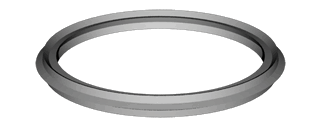Limiting the shapes that could be described only to built-in paths that traced around the original path would be rather restricting on a parametric shape. Thus, I added an additional shape that allowed the user to describe a complex shape using S-Expressions once again to define now the second path. While the S-Expression parser requires that the variable be called x, it must be remembered that this variable is separate from the one used in the original path. In the following example two shapes are made by rotating a polynomial around an unvarying point. The description for the left shape is given.
 ```Geometry "parametric" "string x" "0" "string y" "0" "string z" "0" "string dx" "mult -1 (sin (mult 2 (mult x pi)))" "string dy" "cos (mult 2 (mult x pi))" "string dz" "0" "string shape" "complex" "string cx" "sub 1 (pow x 3)" "string cy" "x"```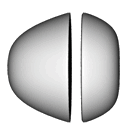There are more parameters for the shape that may be set, if so desired. These include the minimum and maximum values (i.e. the range) of the variable for either of the two paths, the number of samples to take for either of the two paths, whether or not a smoothing should occur between the samples, and two other parameters that can be expressed as S-Expressions. The last two parameters are the radius and twist of the path being traced around the original path. These expressions are expressed in terms of the variable on that first path. Two examples of using these parameters as non-constants are shown below:
 ```Geometry "parametric" "string x" "0" "string y" "0" "string z" "0" "string dx" "mult -1 (sin (mult 2 (mult x pi)))" "string dy" "cos (mult 2 (mult x pi))" "string dz" "0" "string radius" "add 0.2 (mult 0.1 (cos (mult 20 (mult x pi))))"``````Geometry "parametric" "string x" "0" "string y" "0" "string z" "0" "string dx" "mult -1 (sin (mult 2 (mult x pi)))" "string dy" "cos (mult 2 (mult x pi))" "string dz" "0" "string shape" "star" "string radius" "0.2" "string twist" "cos (mult 2 (mult x pi))"```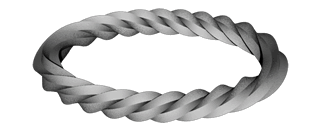Parametric Lights
Parametric lighting requires only the original path to be defined. Samples are then taken along the path and treated as point lights at those sampled points. The intensity of the light is divided by the number of samples taken (optionally set by the integer 'samples' parameter). The following picture shows the use of a parametric shape and light described to render a neon sign sitting in front of a black backdrop. The description of the shape in the front is duplicated for the light source sitting behind it. A distant light illuminates the front shape, while the parametric light adds its illumination to the backdrop. The picture on the left is a photograph of a neon light (taken from the web, possibly doctored a bit) and that on the right is my rendering.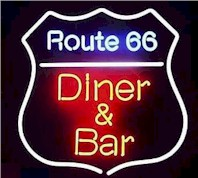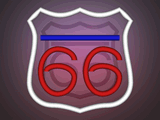Final Image

I constructed a final image that shows off how complex shapes can be created quite easily using mathematical formulas in parametric shapes. With the exception of the walls, table, and spherical light source, all of the objects in this scene are created using my parametric shape. Both the fluorescent light and the basket show how compactly a complex shape could be described that would require a huge amount of effort to create using NURBS. The plastic attachment to the fluorescent light demonstrates the parametric shape where two variables are used. The spines on the tower of hanoi show how boolean logic and math functions can be combined on the radius to make it simple to define a cylinder with varying radius. The tower also shows how primitive shapes like disks and boxes can also be described parametrically. (Click on the image to see it in larger form)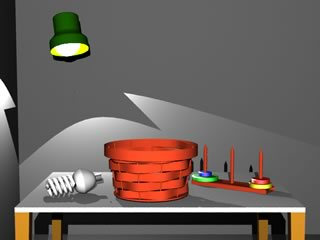Source Code & SCENE Files
The following link provides a zipped up file that contains the scenes used to demonstrate the parametric object as well as the source code needed to extends lrt. There are two source code files: sexpr.h and parametric.cc. Both belong in lrt's shapes directory. The Makefile.filelist needs also to be modified to include parametric.cc as a shape source code file. The parametric.cc file is a large file because it includes some of the other shape code files in it, namely trianglemesh and subdivision. I also created a power point presentation that describes this project which is linked to bellow.